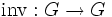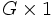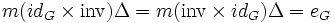# Group object

## Definition

The notion of group object is a category-theoretic generalization of the concept of group. The definition is designed so that a group object in the category of sets is just a group.

Let$C$ be a category with a terminal object$1$ and which allows for taking finite products. A group object over$C$ is an object$G$ is$C$ endowed with the following additional structures:

Structure name Structure description Interpretation when$C$ is the category of sets
multiplication map a morphism$m: G \times G \to G$ where$G \times G$ is the product in the category$C$ ($m$ is sometimes denoted$\mu$)$m$ is a binary operation from the cartesian product$G \times G$ to$G$. In the concrete category case, the multiplication map may be denoted using an infix operator such as$*$ or even simply by concatenation, as with the usual notion of groups.
identity element a morphism$e:1 \to G$ a chosen element of$G$ (which plays the role of identity element). The element itself is denoted$e$ or$1$>
inverse map a morphism$\operatorname{inv}:G \to G$ ($\operatorname{inv}$ is sometimes denoted as$\iota$).$\operatorname{inv}$ is a unary map from the set$G$ to itself. In the concrete category case, the inverse map may be denoted by the superscript${}^{-1}$, as with the usual notion of groups.

satisfying the following compatibility conditions

Condition name Description of condition (verbal) Description of condition (commutative diagram) Interpretation when$C$ is the category of sets
Associativity$m(m\times id_G) = m(id_G \times m)$.Here,$id_G$ denotes the identity map from$G$ to itself. PLACEHOLDER FOR INFORMATION TO BE FILLED IN: [SHOW MORE] In this case, it just becomes the associativity condition on elements:$(a * b) * c = a * (b * c)$ for all$a,b,c \in G$.
Identity element$m(id_G \times e) = p_1$ where$p_1$ is the canonical projection from$G \times 1$ to$G$$m(e \times id_G) = p_2$ where$p_2$ is the canonical projection from$1 \times G$ to$G$
PLACEHOLDER FOR INFORMATION TO BE FILLED IN: [SHOW MORE]$a * e = e * a = a$ for all elements$a\in G$.
Inverse if$\Delta:G \to G \times G$ is the diagonal map (see definition below) and$e_G:G \to G$ is$e$ composed with the unique map from$G$ to$1$ then$m(id_G \times \operatorname{inv})\Delta = m(\operatorname{inv} \times id_G)\Delta = e_G$ PLACEHOLDER FOR INFORMATION TO BE FILLED IN: [SHOW MORE]$a * a^{-1} = ^{-1} * a = e$ for all elements$a \in G$.

The diagonal map$\Delta$ is the unique map$G \to G \times G$ which, when post-composed with the projection map to either factor$G$, gives the identity map from$G$ to$G$.

## Facts

### Group objects in a category form a new category

For any category$C$ that has products and a terminal object, the group objects in$C$ form a category with a forgetful functor to$C$. The category is described explicitly as follows:

• The objects of this category are the group objects in$C$.
• A morphism in this category between two group objects in$C$ is a morphism between them as objects of$C$ that commutes with the group operations.

The forgetful functor simply sends a group object in$C$ to its underlying object in$C$, and views morphisms as$C$-morphisms. It is a faithful functor.

### Group objects and functors between categories

Suppose$C_1$ and$C_2$ are categories and$F:C_1 \to C_2$ is a functor. It is tempting to believe that$F$ induces a functor from the category of group objects of$C_1$ to the category of group objects of$C_2$. This is true if the functor$F$ sends the terminal object to the terminal object and sends products to products (in other words, it is a monoidal functor between the categories equipped with the Cartesian monoidal structure).

An example of the failure of this is that algebraic groups are not topological groups. The functor from algebraic varieties over a field (such as$\mathbb{C}$) to topological spaces that sends any algebraic variety to its underlying set with the Zariski topology. This functor does not preserve products, i.e., the Zariski topology on a product variety is not the product topology of the Zariski topologies on the varieties.

## Particular cases

Category (described by name for its typical object) Notion of group object
group abelian group (this is a corollary of the Eckmann-Hilton argument)
topological space topological group
algebraic variety over a fixed (usually, algebraically closed) field algebraic group over the same field
manifold real Lie group
differential manifold real Lie group
simplicial set simplicial group
cosimplicial set cosimplicial group